Coming back to the third goal, it is to determine the value of terminal market of the firm. This is the value which gives the value of the firm which can be sold for in the future at time T which can be calculated by the growing pertuity formula as follows: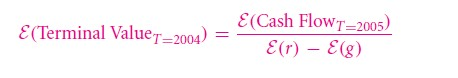Let’s reply the remainder on the cash flow forecast which is direct from the table 202. But here, you will still require the estimates for the growth rate of the company eventually.

Let’s consider the cost of future of capital initially:

20.5 A the Cost of Capital

One can rely on the CAPM in order to determine capital cost for the company Pepsi Co for the year late 2001. If the company is not listed in publicly traded, you can use the data from the comparable company like Coca-Cola. Table 20.5 includes the prices of stock on the company Yahoo, Pepsi Co. and Coca Cola. In order to determine the rate of return in the past, you can use this table to obtain the prices as mentioned below: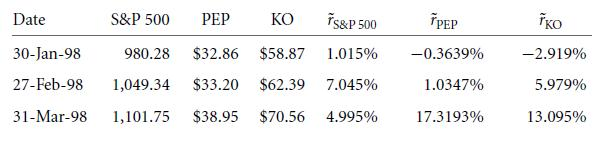(Considering an example: r = 1,101.75/1,049.34 − 1 ≈ 4.995). With the calculated return rate, one can now determine the required statistics from the historical data: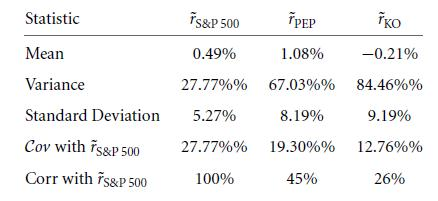This list of the statistical data will help you with the calculation of equity beta from historical data of PepsiCo and Coca-Cola:

Now the question here is which estimate is the best one supporting the future equity beta of Pepsi Co?

• Consider 0.7 as the historical equity beta of Pepsi Co
• Or you will consider that the beta value must be shrunken towards the beta average βM,M = 1 in market. This case will reflect the market beta as = (7 + 1)/2 = 0.85.
• You will look for industrial beta which is more reliable and trustable than Pepsi Co own beta as they rely on the historical outlay of a single firm.
• Or you make an assumption that Coca-Cola and Pepsi Co are similar companies, which means you will choose the value of market beta as 0.46 as an average figure between both the companies which means that the best estimate of beta value will be 0.6.

In order to consider CAPM, one needs to estimate the rate of economy wide which is risk free along with premium of equity:

The risk-free rate: The treasury yield of 5-year and 20 year was calculated as 4.4% and 5.7% in the year end of 2001 respectively. So one can choose 10 years as the rate of interest for Pepsi Co which means rate is 5% per year approximately.

The Equity Premium: Let’s consider that the board members of Pepsi Co. along with management have declared 3% as per the standard estimate.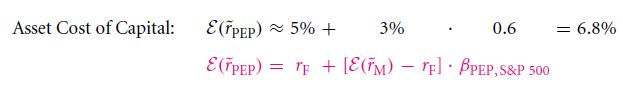So let’s consider this as 7%. This means that estimate of the capital cost for Pepsi Co. is between 5 to 10%.

20.5 B the Cost of Capital minus the Growth Rate of cash Flows

You are still in need of an estimation of growth rate of cash flows under expected eternal (E(g)) for the perpetuity formula or we can say, capital cost E(r) subtracted by this rate of growth. One can notice that it is easier to assume the upper bounds which are high on the sustaining rate of growth which is true for lower bounds too.

But a company like Pepsi CO. is hardly to experience lower bound but one can assume the same in order to realize the real rate of growth.

But you need some additional information to estimate the same. The following resources will help you with the required information:

1. Internal Company information: Getting the internal information from a company like Pepsi CO, can be really tough. But the publically traded information can value at some extend. But if you are an internal analyst of the firm, you can enjoy the ease of internal information easily.
2. Industry or comparable firm information: If you are lucky to get information on the estimate growth rate of competitors like Coca-Cola, the process becomes simpler.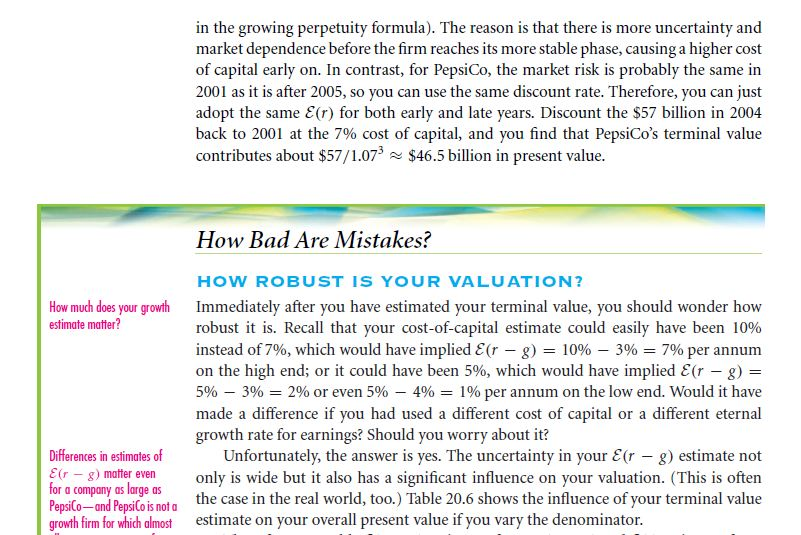Links of Next Financial Accounting Topics:-### Customer Reviews

My Homework Help
Rated 5.0 out of 5 based on 510 customer reviews at